## [ITOC-ch5] REDUCIBILITY

If A reduces to B

• we can use a solution to B to solve A
• solving A is no harder than solving B

$A \longrightarrow B \ (decidable) \Rightarrow A \ (decidable)$

$A \ (undecidable) \longrightarrow B \Rightarrow B \ (undecidable)$

### 5.1 UNDECIDABLE PROBLEMS FROM LANGUAGE THEORY

General Idea

• Contradiction on $A \longrightarrow B \ (decidable) \Rightarrow A \ (decidable)$

• If we have a TM R that decides $HALT_{TM}$ ( or sth ).

• Then we can use R to construct a TM S that decides $A_{TM}$

$A_ {TM} ≤m HALT{TM}$

$HALT_{TM} = \{ < M, w> \ | \ M \ is \ a \ TM \ and \ M \ halts \ on \ input \ w \}.$

Proof

If M loop on w, we can use R to reject.

S = “On input $<M, w>$, an encoding of a TM M and a string w:

1. Run TM R on input $<M, w>$.
2. If R rejects, reject .
3. If R accepts, simulate M on w until it halts.
4. If M has accepted, accept ; if M has rejected, reject .”

$A_ {TM} ≤m E{TM}$

$E_{TM} = \{ < M >| \ M \ is \ a \ TM \ and \ L(M) = ∅ \}.$

Proof

If $L (M_1)$ is empty, than M accept w

S = “On input $<M, w>$, an encoding of a TM M and a string w:

1. Construct $M_1$ = “On input x:
1. If $x \neq w$, reject .
2. If $x = w$, run M on input w and accept if M does.”
2. Run R on input $< M_1 >$.
3. If R accepts, reject ; if R rejects, accept .”

$A_ {TM} ≤m REGULAR{TM}$

$REGULAR_{TM} = \{ < M >| \ M \ is \ a \ TM \ and \ L(M) \ is \ a \ regular \ language \}.$

Proof

If $L(M_2)$ is regular, than M accept w

If M does not accept w

• $M_2$ recognize $\{0^n 1^n \ | \ n ≥ 0 \}$ which is nonregular

If M accepts w

• $M_2$ recognize all strings $Σ^ *$ ( including $0^n 1^n$) which is regular

S = “On input $<M, w>$, where M is a TM and w is a string:

1. Construct the following TM $M_2$ .
$M_2$ = “On input x:
• If x has the form $0^n 1^n$ , accept .
• If x does not have this form, run M on input w and accept if M accepts w.”
2. Run R on input $<M_2 >$.
3. If R accepts, accept; if R rejects, reject .”

Rice’s theorem

• determining any property of the languages recognized by Turing machines is undecidable.

$E_ {TM} ≤m EQ{TM}$

$EQ_{TM} = \{ < M_1 , M_2 > | \ M_1 \ and \ M_2 \ are \ TMs \ and \ L(M_1) = L(M_2) \}.$

Proof

$M_1$ is a TM that rejects all inputs.

If $L(M) = L(M_1)$, than $L(M) = ∅$

REDUCTIONS VIA COMPUTATION HISTORIES

computation history

• the sequence of configurations that the machine goes through as it processes the input
• Finite sequences. If M doesn’t halt on w, no accepting or rejecting computation history exists for M on w.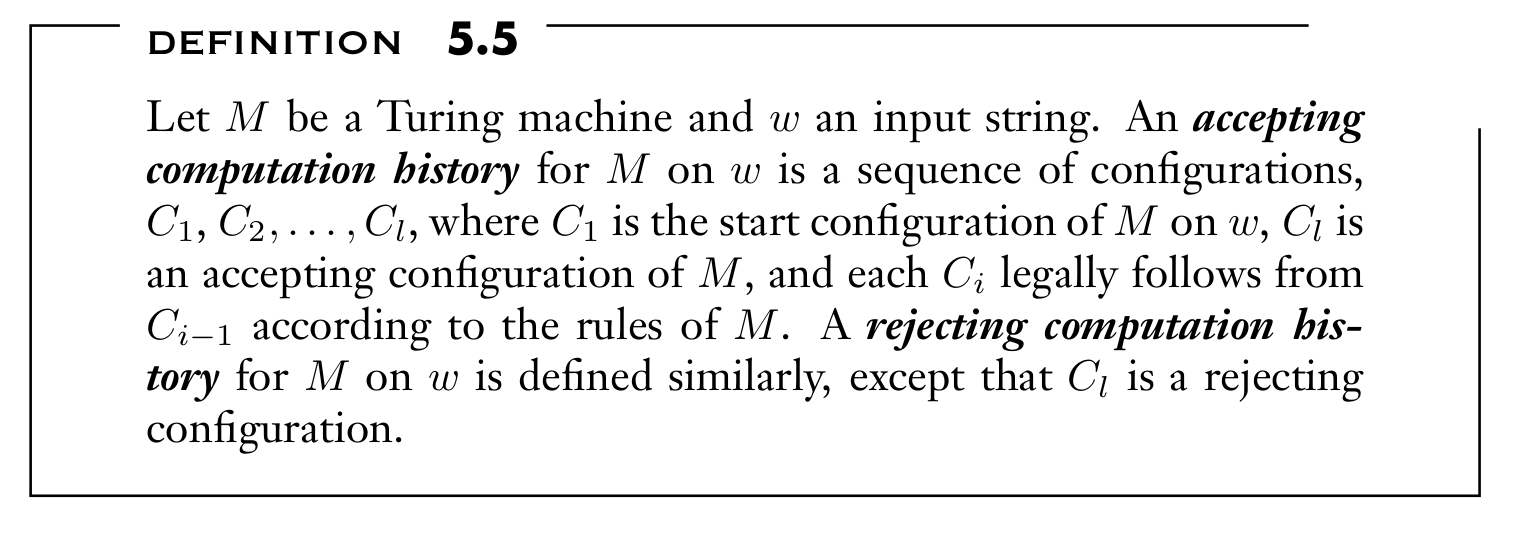Linear bounded automaton

• the amount of memory is limited and linear in input size n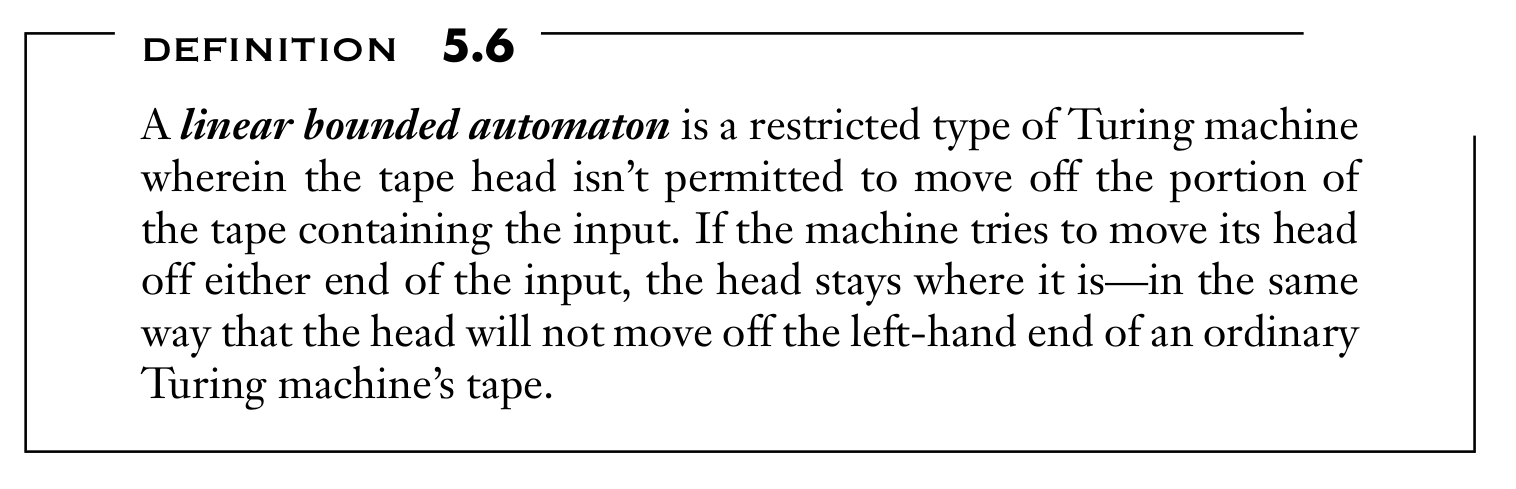decidable

$A_{LBA} = \{ < M, w>| \ M \ is \ an \ LBA \ that \ accepts \ string \ w \}.$

LEMMA 5.8

• If a LBA has q states and g symbols with tape length n.
• There are exactly $qng^n$ distinct configurations.

Proof

• reject after $qng^n$ steps because M is looping

$A_ {TM} ≤m E{LBA}$

$E_{LBA} = \{ < M >| \ M \ is \ an \ LBA \ where \ L(M) = ∅ \}.$

Proof

If $L(B) \neq ∅$ = { all accepting computation histories for M on w }, than M accepts w.

Construct B to generate all strings that

1. $C_1$ is the start configuration for M on w.
2. $C_l$ is an accepting configuration for M .
3. Each $C_{i+1}$ legally follows from $C_i$ .

$A_ {TM} ≤m ALL{LBA}$

$ALL_{CFG} = \{ < G >| \ G \ is \ a \ CFG \ and \ L(G) = Σ ^ ∗ \}.$

Proof

If $L(G) \neq Σ ^ ∗$ = { all strings except all computation histories for M on w }, than M accepts w.

Construct G to generate all strings that

1. do not start with $C_1$
2. do not end with an accepting configuration
3. some $C_i$ does not properly yield $C_{i+1}$ under the rules of M

### 5.2 A SIMPLE UNDECIDABLE PROBLEM

an example of an undecidable problem not concerning automaton

Post Correspondence Problem

• determine that a collection of dominos has a match (repetitions permitted).$A_ {TM} ≤_m PCP$

$PCP = \{ < P >| \ P \ is \ an \ instance \ of \ the \ Post \ Correspondence \ Problem \ with \ a \ match\}.$

Proof

If the P we construct has a match, than M accepts w

Construct P

• let P be an instance of MPCP that starting matching with the first domino.

• begins with configuration $C_1$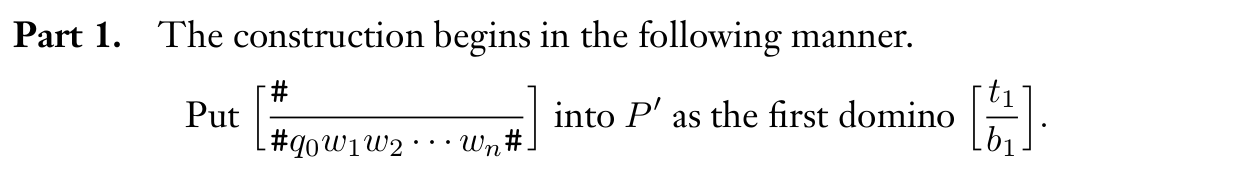• Head motions to right and left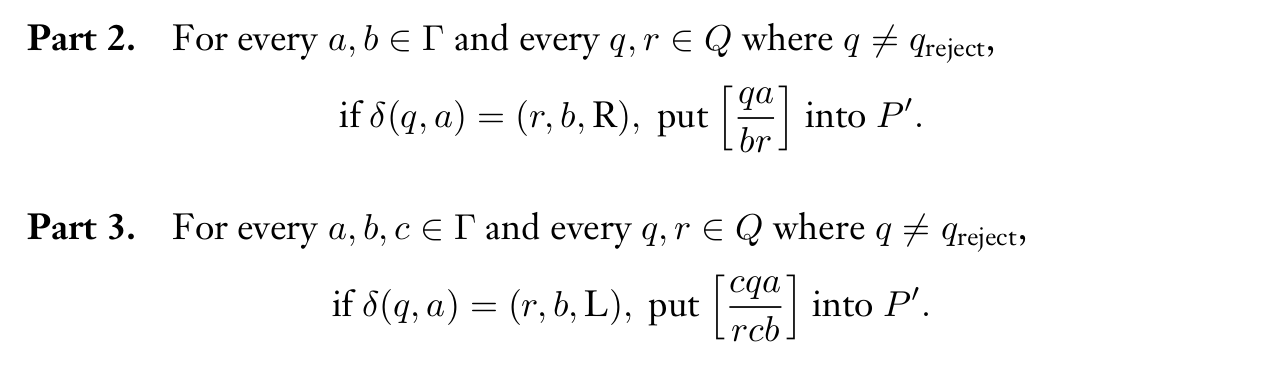• Other tape alphabet surrounded the head• Separation of the configuration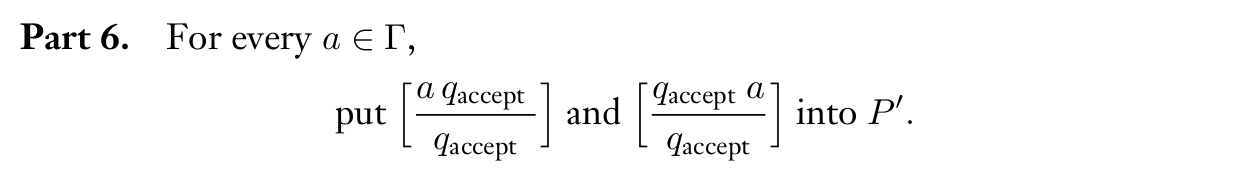• Final alignment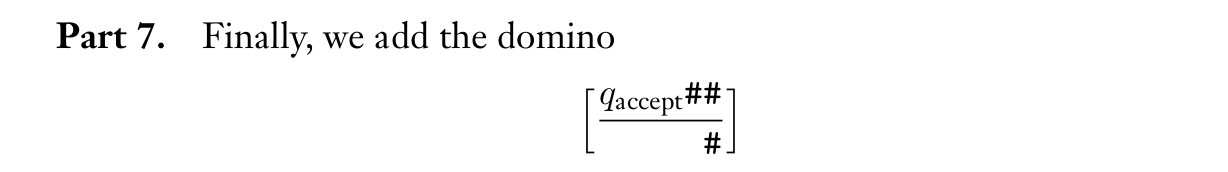• add * symbol so that the only possible match is start with the first domino. ( change MPCP into PCP )### 5.3 MAPPING REDUCIBILITY

formalize the notion of mapping reducibility

COMPUTABLE FUNCTIONS

f == TM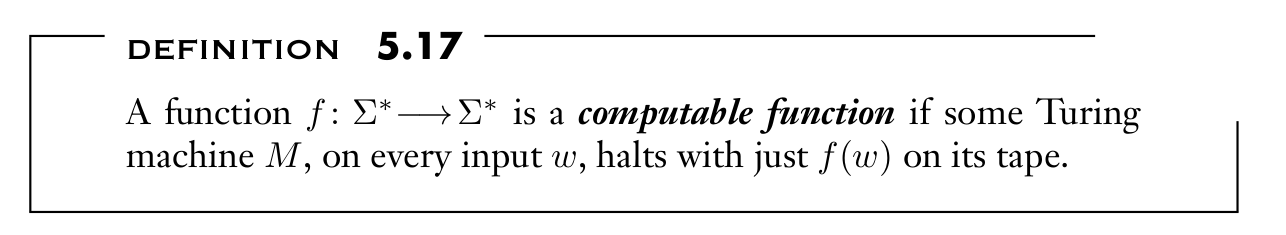FORMAL DEFINITION OF MAPPING REDUCIBILITY

mapping reducibility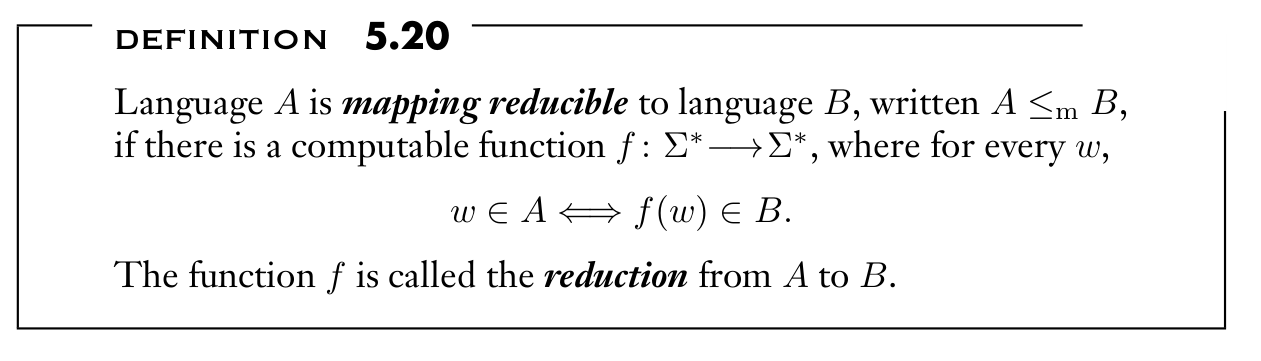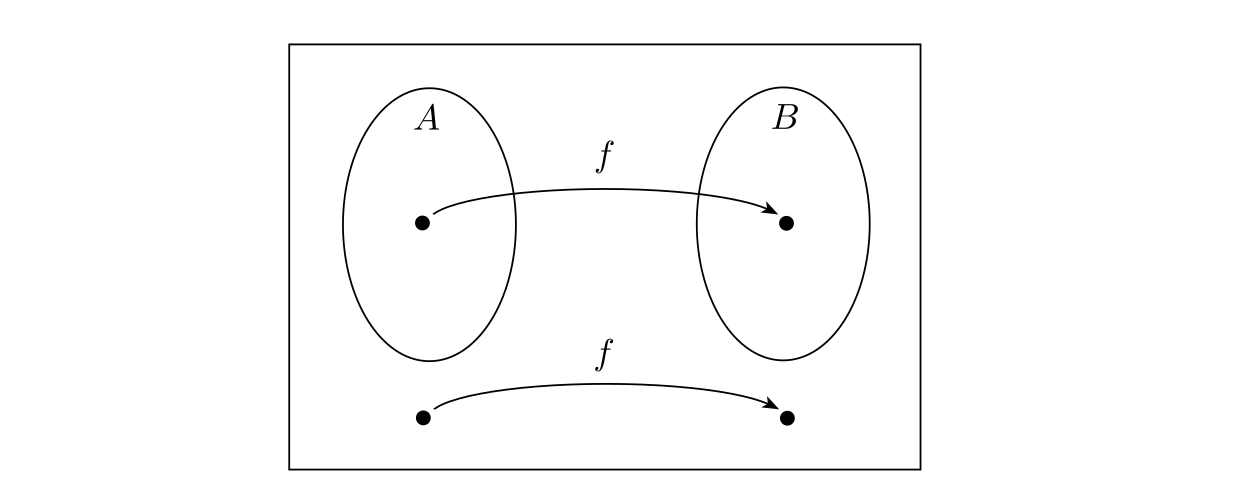If $A ≤_m B$ and B is decidable, then A is decidable.

Proof

construct A’s decider N = “On input w:

1. Compute f (w).
2. Run M on input f (w) and output whatever M outputs.”

If $A ≤_m B$ and A is undecidable, then B is undecidable.

$A \ (undecidable) \longrightarrow B \Rightarrow B \ (undecidable)$

Example of computable function

• Input $< M,w >$ while output 
• $< M, w > ∈ A_{TM}$ if and only if $∈ HALT_{TM}$.

(5.1中,在每个S里构造的机器就是这里的reduce function)

If $A ≤_m B$ and B is Turing-recognizable, then A is Turing-recognizable.

If $A ≤_m B$ and A is not Turing-recognizable, then B is not Turing-recognizable.

if $A_{TM} ≤m \overline{B}$ then $\overline{A{TM}} ≤_m B$ therefore B isn’t recognizable.

$EQ_{TM}$ is neither Turing-recognizable nor co-Turing-recognizable.

Proofed by corollary above.